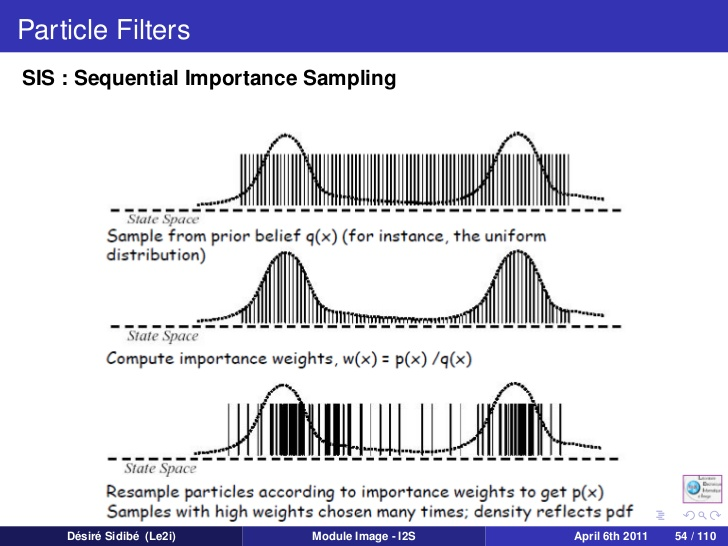Importance sampling is related to rejection sampling, which I looked at in the last post. Here is a short demo.

This file contains bidirectional Unicode text that may be interpreted or compiled differently than what appears below. To review, open the file in an editor that reveals hidden Unicode characters.

 %% true probability distribution true_func = @(x) betapdf(x,1+1,1+10); %% Do importance sampling N = 10^6; % uniform proposal distribution x_samples = rand(N,1); proposal = 1/N; % evaluate for each sample target = true_func(x_samples); % calculate importance weight w = target ./ proposal; w = w ./ sum(w); % resample, with replacement, according to importance weight samples = randsample(x_samples,N,true,w); %% plot subplot(1,2,1) x = linspace(0,1,1000); plot(x, true_func(x) ) axis square subplot(1,2,2) hist(samples,1000) title('importance sampling') axis square

A problem of rejection sampling is that many samples could be evaluated in regions of low probability mass. This then lead to a high rate of attrition, with many samples being rejected. In importance sampling, this seems like less of an issue in terms of ending up with a large number of samples for an accurate representation of the distribution. Although the same basic problem is there in that the probability is being evaluated for many points in parameter space with very low or zero probability.So let’s do the same thing from the last post and use this to do parameter estimation.

This file contains bidirectional Unicode text that may be interpreted or compiled differently than what appears below. To review, open the file in an editor that reveals hidden Unicode characters.

 true_mean = 0; true_sigma = 1; % likelihood_func = @(x, mean, sigma) normpdf(x, mean, sigma); % the above function to calcalate in matrix form, for speed likelihood_func = @(x, mean, sigma)… prod(normpdf(repmat(x,[1 numel(mean)]),… repmat(mean, [1 numel(x)])',… repmat(sigma,[1 numel(x)])' ), 1); %% generate data N=20; observed_data = normrnd(true_mean, true_sigma, [N 1]); %% Do importance sampling % create many samples for mean and sigma N = 10^6; mean_samples = (rand(N,1)-0.5)*5; sigma_samples = rand(N, 1) * 10; proposal = 1/N; % evaluate likelihood for each (mean, sigma) sample target = likelihood_func(observed_data, mean_samples, sigma_samples); % calculate importance weight w = target ./ proposal; w = w ./ sum(w); % resample, with replacement, according to importance weight sample_ind = randsample([1:N],N,true,w); mean_samples = mean_samples(sample_ind); sigma_samples = sigma_samples(sample_ind); %% plot plot(mean_samples,sigma_samples,'.') xlabel('mean') ylabel('sigma') hold on plot(true_mean, true_sigma, 'r.','MarkerSize',5^2) axis square

Which results in thisHere is a nice little figure I found that helped with the intuition.## Join the conversation

1.2.1.John says: